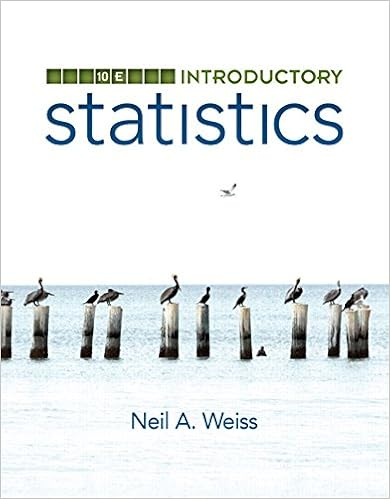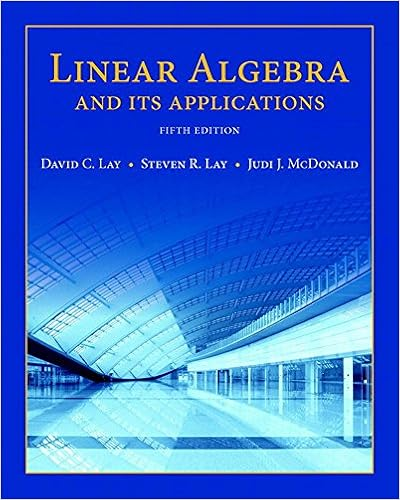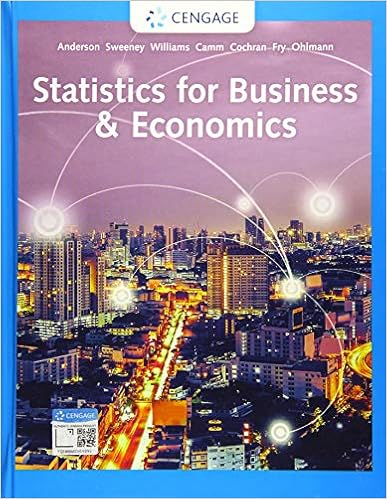# Taylornotes - Estimation of functions using Taylor...

• 34

This preview shows page 1 - 4 out of 34 pages.

The preview shows page 2 - 4 out of 34 pages.
Estimation of functions using Taylor polynomialscFrank Zorzitto, Faculty of Mathematics, University of WaterlooIf a power seriesa0+a1(x-p) +a2(x-p)2+· · ·+ak(x-p)k+. . .converges to a functionfon some interval(p-R, p+R)centered atp, then theseries’ coefficientsakmust be the Taylor coefficients off. Namely,ak=f(k)(p)k!,wheref(k)denotes the derivative offtakenktimes.This prompts us to pose the converse question. If a functionfhas derivatives ofall ordersf(k)(x), wherexruns through some interval(p-R, p+R), must theresulting Taylor seriesf(p) +f0(p)(x-p) +f00(p)2(x-p)2+· · ·+f(k)(p)k!(x-p)k+. . .converge tof(x)for allxin(p-R, p+R)?In order to answer our question, we need to examine theerrorthat the partial sumsof the Taylor series forfmake in estimatingf(x).The Taylor polynomialWe start with a functionfdefined on an intervalIthat contains a numberp. Sup-pose that the firstnderivatives off, namelyf0, f00, f000, . . . , f(n), are also all de-fined. There is no shortage of such functions. For every one of thesef, the poly-nomialTn(x) =f(p) +f0(p)(x-p) +f00(p)2!(x-p)2+· · ·+f(n)(p)n!(x-p)nis called theTaylor polynomial off, of degree up ton, expanded aboutp.As we observed at the start, iffis represented by a power seriesf(x) =a0+a1(x-p)+a2(x-p)2+· · ·+ak(x-p)k+. . .on some interval aroundp,1
##### We have textbook solutions for you!The document you are viewing contains questions related to this textbook.
Chapter 16 / Exercise 14
Calculus
StewartExpert VerifiedBrowse all Textbook Solutions
then then’th partial sum of this series is nothing but the Taylor polynomial offofdegree up ton.In sigma-notation, the Taylor polynomial can also be written asTn(x) =nXk=0f(k)(p)k!(x-p)k.The numbersf(k)(p)k!that go with(x-p)kare called theTaylor coefficientsoffat the pointp.We start counting our Taylor polynomials atn= 0.Whenn= 0, the Taylorpolynomial of degree up to0is the constant polynomialT0(x) =f(p).Whenn= 1, the Taylor polynomial is the much more interesting linear functionT1(x) =f(a) +f0(a)(x-a).Of course,T1(x)is the well known tangent line, also known as thelinear approxi-mationor thelinearization, offatp. Whenn= 2, the second Taylor polynomialbecomesT2(x) =f(p) +f0(p)(x-p) +f00(p)2(x-p)2.Whenn= 3we getT3(x) =f(p) +f0(p)(x-p) +f00(p)2(x-p)2+f000(p)6(x-p)3,and so on.The Taylor polynomial is special forfbecauseit is the one and only polynomial ofdegree up tonthat agrees withfand all of the derivativesf0, f00, . . . , f(n)at thecentral pointp.That isTn(p) =f(p)T0n(p) =f0(p)T00n(p) =f00(p)...T(n)n(p) =f(n)(p).2
Let us demonstrate this key observation forn= 2. A general polynomial of degreeup to2can be written to look likeg(x) =r+s(x-p) +t(x-p)2,wherer, s, tare the coefficients.We can see quite readily thatg0(x) =s+ 2t(x-p),g00(x) = 2t,and thusg(p) =r, g0(p) =s,g00(p) = 2t.Then the requirementsg(p) =f(p), g0(p) =f0(p), g00(p) =f00(p)come down to saying thatr=f(p),s=f0(p),t=f00(p)/2which means precisely thatg(x) =f(p) +f0(p)(x-p) +f00(p)2(x-p)2.

Course Hero member to access this document

Course Hero member to access this document

End of preview. Want to read all 34 pages?

Course Hero member to access this document

Term
Fall
Professor
SPEZIALE
Tags
dx, Taylor Polynomial
##### We have textbook solutions for you!
The document you are viewing contains questions related to this textbook.The document you are viewing contains questions related to this textbook.
Chapter 16 / Exercise 14
Calculus
StewartExpert Verified
•••